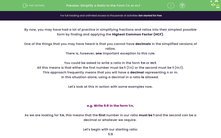# Simplify a Ratio to the Form 1:n or m:1

In this worksheet, students will create and simplify ratios in the form 1:n or m:1 where the values of m and n can be decimals, converting values into the same units where required.Key stage:  KS 4

Year:  GCSE

GCSE Subjects:   Maths

GCSE Boards:   AQA, Eduqas, Pearson Edexcel, OCR,

Curriculum topic:   Ratio, Proportion and Rates of Change

Curriculum subtopic:   Ratio, Proportion and Rates of Change Calculations with Ratio

Difficulty level:#### Worksheet Overview

By now, you may have had a lot of practice in simplifying fractions and ratios into their simplest possible form by finding and applying the Highest Common Factor (HCF).

One of the things that you may have heard is that you cannot have decimals in the simplified versions of ratios.

There is, however, one important exception to this rule.

You could be asked to write a ratio in the form 1:n or m:1.

All this means is that either the first number must be 1 (1:n) or the second must be 1 (m:1).

This approach frequently means that you will have a decimal representing n or m.

In this situation alone, using a decimal in a ratio is allowed.

Let's look at this in action with some examples now.

e.g. Write 5:8 in the form 1:n.

As we are looking for 1:n, this means that the first number in our ratio must be 1 and the second can be a decimal or whatever we require.

Let's begin with our starting ratio:

5:8

In order to convert the first number to 1, we have to divide by 5 (as 5 ÷ 5 = 1).

So we need to do the same thing to the second number in the ratio:

5:8 ÷ 5 = 1 : 1.6

e.g. Write 25:10 in the form m:1.

As we are looking for m:1, this means that the second number in our ratio must be 1 and the first can be a decimal or whatever we require.

In order to convert the second number to 1, we have to divide by 10 (as 10 ÷ 10 = 1).

25:10 ÷ 10 = 2.5 : 1

In this activity, we will create and simplify ratios in the form 1:n or m:1 where the values of m and n can be decimals, converting values into the same units where required.

### What is EdPlace?

We're your National Curriculum aligned online education content provider helping each child succeed in English, maths and science from year 1 to GCSE. With an EdPlace account you’ll be able to track and measure progress, helping each child achieve their best. We build confidence and attainment by personalising each child’s learning at a level that suits them.

Get started